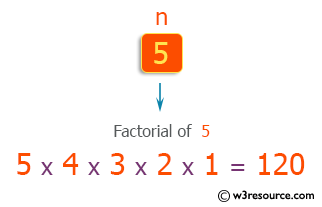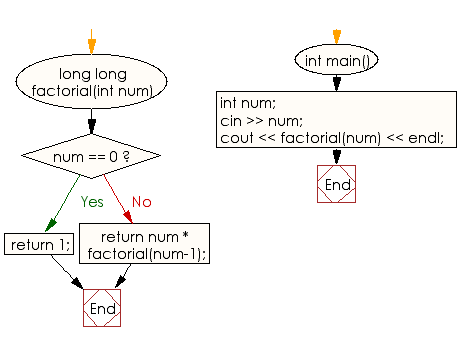﻿ C++ Exercises: Read an integer n and prints the factorial of n - w3resource

# C++ Exercises: Read an integer n and prints the factorial of n

## C++ Basic: Exercise-69 with Solution

For n = 10, write a C++ program that reads the integer n and prints its factorial.

Pictorial Presentation:Sample Solution:

C++ Code :

``````#include <iostream>
using namespace std;

long long factorial(int num) {
if (num == 0) {
return 1;
}
else {
return num * factorial(num-1);
}
}

int main() {
int num;
cin >> num;
cout << factorial(num) << endl;
}
``````

Sample Output:

```sample Output
1
```

Flowchart:C++ Code Editor:

What is the difficulty level of this exercise?

﻿

## C++ Programming: Tips of the Day

Why is there no std::stou?

The most pat answer would be that the C library has no corresponding "strtou", and the C++11 string functions are all just thinly veiled wrappers around the C library functions: The std::sto* functions mirror strto*, and the std::to_string functions use sprintf.

Ref: https://bit.ly/3wtz2qA

We are closing our Disqus commenting system for some maintenanace issues. You may write to us at reach[at]yahoo[dot]com or visit us at Facebook